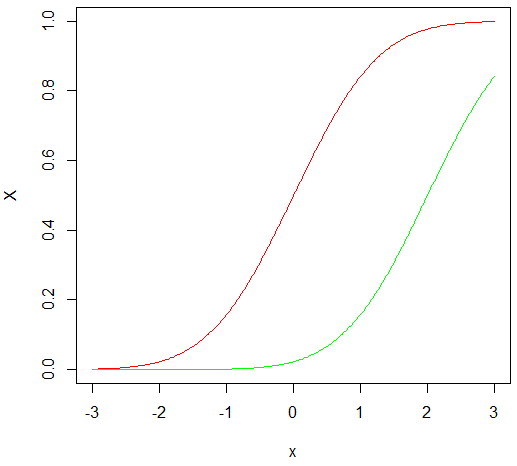# How to create two line charts in the same plot in R?

We can do this by using lines function after plotting the first chart.

## Example

> x <- seq(-3, 3, 0.025)
> X1 <- pnorm(x)
> X2 <- pnorm(x, 2, 1)
> plot(x, X1, type="l",col="red", ylab="X")
> lines(x, X2, col="green")